Atmos. Chem. Phys., 21, 6035–6051, 2021
https://doi.org/10.5194/acp-21-6035-2021
Atmos. Chem. Phys., 21, 6035–6051, 2021
https://doi.org/10.5194/acp-21-6035-2021

Research article 22 Apr 2021

Research article | 22 Apr 2021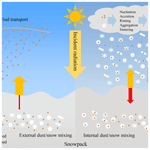# Enhanced light absorption and reduced snow albedo due to internally mixed mineral dust in grains of snow

Enhanced light absorption and reduced snow albedo due to internally mixed mineral dust in grains of snow
Tenglong Shi1, Jiecan Cui1, Yang Chen1, Yue Zhou1, Wei Pu1, Xuanye Xu2, Quanliang Chen2, Xuelei Zhang3, and Xin Wang1,4 Tenglong Shi et al.
• 1Key Laboratory for Semi-Arid Climate Change of the Ministry of Education, College of Atmospheric Sciences, Lanzhou University, Lanzhou 730000, China
• 2College of Atmospheric Sciences, Chengdu University of Information Technology, Chengdu 610225, China
• 3Key laboratory of Wetland Ecology and Environment, Northeast Institute of Geography and Agroecology, Chinese Academy of Sciences, Changchun 130102, China
• 4Institute of Surface-Earth System Science, Tianjin University, Tianjin 300072, China

Correspondence: Xin Wang (wxin@lzu.edu.cn)

Abstract

Mineral dust is a major light-absorbing aerosol, which can significantly reduce snow albedo and accelerate snow/glacier melting via wet and dry deposition on snow. In this study, three scenarios of internal mixing of dust in ice grains were analyzed theoretically by combining asymptotic radiative transfer theory and (core–shell) Mie theory to evaluate the effects on absorption coefficient and albedo of the semi-infinite snowpack consisting of spherical snow grains. In general, snow albedo was substantially reduced at wavelengths of <1.0µm by internal dust–snow mixing, with stronger reductions at higher dust concentrations and larger snow grain sizes. Moreover, calculations showed that a nonuniform distribution of dust in snow grains can lead to significant differences in the values of the absorption coefficient and albedo of dust-contaminated snowpack at visible wavelengths relative to a uniform dust distribution in snow grains. Finally, using comprehensive in situ measurements across the Northern Hemisphere, we found that broadband snow albedo was further reduced by 5.2 % and 9.1 % due to the effects of internal dust–snow mixing on the Tibetan Plateau and North American mountains. This was higher than the reduction in snow albedo caused by black carbon in snow over most North American and Arctic regions. Our results suggest that significant dust–snow internal mixing is important for the melting and retreat of Tibetan glaciers and North American mountain snowpack.

Share
1 Introduction

Snow cover is one of the most reflective surfaces in the Earth system and plays a crucial role in the atmospheric solar radiation energy budget via snow–albedo feedbacks (Di Mauro et al., 2020; Flanner et al., 2011; Jacobson, 2004; Usha et al., 2020; Xie et al., 2018). Previous studies have shown that light-absorbing particles (LAPs) effectively reduce snow albedo and enhance the absorption of solar radiation after deposition. These studies were based on in situ observations and model simulations (Casey et al., 2017; Hadley and Kirchstetter, 2012; Shi et al., 2020; Warren and Wiscombe, 1980; Yasunari et al., 2011, 2015). As a result, snow contaminated with LAPs shows significant changes in morphology (Niwano et al., 2012; Rango et al., 1996), chemistry (France et al., 2012; Reay et al., 2012), hydrology (Matt et al., 2018; Qian et al., 2015; Rahimi et al., 2019), snowmelt rate (Kaspari et al., 2015; Warren, 1984), and radiative properties (Grenfell et al., 2002; Hansen and Nazarenko, 2004; Zhao et al., 2014).

Numerous studies have assessed the potential effects of LAPs, such as black carbon (BC) and mineral dust, on snow albedo by assuming that LAPs mixed outside spherical snow grains (i.e., external mixing) (Flanner et al., 2007; Kokhanovsky, 2013; Libois et al., 2013; Wang et al., 2017; Warren and Wiscombe, 1980). For example, Warren and Wiscombe (1980) calculated snow spectral albedo by solving a radiative transfer equation using Mie theory and δ-Eddington approximations, and they found that 10–100 ng g−1 of BC and 1–10 µg g−1 of dust in old snow decreased albedo by 1 %–7 % and 2 %–10 % at the 400 nm wavelength, respectively. Flanner et al. (2007) pointed out that the reduction in snow albedo for 1000 ng g−1 BC in snow was 0.045 (0.17) with a 50 (1000) µm snow grain radius (Ref) based on the Snow, Ice, and Aerosol Radiation (SNICAR) radiative transfer model. This model utilizes theory from Wiscombe and Warren (1980) and the two-stream radiative transfer solution from Toon et al. (1989). Wang et al. (2017) developed a Spectral Albedo Model for Dirty Snow (SAMDS) based on asymptotic radiative transfer theory, which is a function of the snow grain radius, LAP (e.g., BC, dust) mixing ratios, and mass absorption coefficients (MACs) of LAPs. Their results revealed that broadband snow albedo decreased 0.03 and 0.003 due to 200 ng g−1 of BC and 2 µg g−1 of dust in snow, respectively, with a Ref of 200 µm. Additionally, recent studies found that snow nonsphericity can interact with LAP–snow mixing, which leads to weaker LAP-induced albedo reductions for nonspherical snow shapes than snow spheres (e.g., Dang et al., 2016; He et al., 2018, 2019).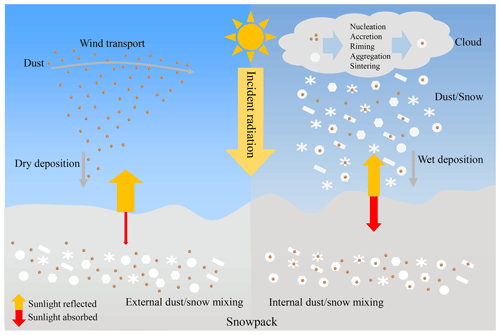Figure 1Schematic of dust mixing with snow grains internally. Dust tends to mix externally with snow grains through dry deposition and/or below-cloud scavenging, while dust–snow internal mixtures can be produced by nucleation, accretion, riming, aggregation, and sintering during aerosol–cloud–precipitation processes known as wet deposition. Arrows represent how the absorption (red) and reflection (yellow) of incoming sunlight changes with dust–snow mixing state.

Recently, direct snowpack observations have shown evidence for the existence of LAP–snow internal mixing (e.g., Horhold et al., 2012; Spaulding et al., 2011). LAPs tend to mix externally with snow grains through dry deposition and/or below-cloud scavenging, while internal LAP–snow mixing can be produced by nucleation, accretion, riming, aggregation, and sintering during aerosol–cloud–precipitation processes (i.e., wet deposition; Fig. 1) (Flanner et al., 2012). Furthermore, Flanner et al. (2012) found that internal BC–ice mixing (IBM) increased the absorption of snowpack by a factor of 1.8–2.1 relative to external BC–ice mixing (EBM). He et al. (2018) indicated that IBM enhanced the mean snow albedo reduction over the Tibetan Plateau by 30 %–60 % relative to EBM, based on the updated SNICAR model. Additionally, Dombrovsky and Kokhanovsky (2020) demonstrated that nonuniform BC distribution in ice grains may lead to significantly different absorption coefficients and snowpack albedo in visible (VIS) wavelengths.

Numerous studies have addressed the role of IBM in enhancing the absorption of snowpack due to its strong absorption effect relative to other LAPs (Dombrovsky and Kokhanovsky, 2020; Flanner et al., 2012; He et al., 2018; Liou et al., 2011). In contrast, few studies have considered the effects of internal dust–ice mixing (IDM) in snowpack. Liou et al. (2014) are pioneers in investigating the dust–snow internal mixing effects based on the geometric-optics surface-wave approach. Subsequently, He et al. (2019) used the same method to explicitly quantify the combined effects of dust–snow internal mixing and snow grain nonsphericity on snow optical properties. Thereafter, they developed a set of new dust–snow parameterizations for land–climate modeling applications for the first time. Actually, dust particles are generally larger than BC and act as more efficient ice nuclei, showing a better ability to influence cloud formation and precipitation (Creamean et al., 2013; Huang et al., 2014). Therefore, they are more likely to mix internally with ice grains. Furthermore, dust can also dominate light absorption and effectively decrease snow albedo because of its relatively high mass abundance (ppm) in snowpack, especially in areas with seasonal and patchy snow cover or mountainous regions (Di Mauro et al., 2015; Gabbi et al., 2015; Painter et al., 2012; Reynolds et al., 2020; Xie et al., 2018). Therefore, it is important to account for IDM when estimating the impact of dust deposition on snow albedo.

In this study, we assess the effects of external and internal mixing of dust with ice grains on the snowpack absorption coefficient and albedo using asymptotic radiative transfer theory and Mie theory. In addition, the uniformity and nonuniformity of dust particle distribution inside ice grains are considered for IDM based on the effective medium approximation, and the combined effects of dust content and snow grain radius on snow albedo are quantified. A schematic of various dust spatial distributions from this study is presented in Fig. 2. We further discuss snow albedo sensitivity to complex refractive indices and dust particle size distribution. Based on a comprehensive set of field measurements of dust concentrations, we estimate the reductions in snow albedo by dust external and internal mixing with ice grains across the Northern Hemisphere.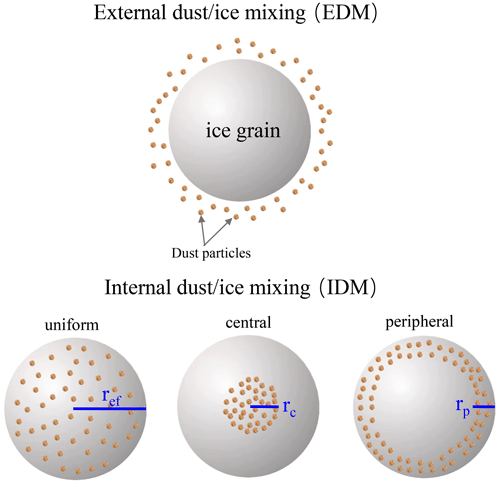Figure 2Schematic depicting various mixing scenarios of snow grains and dust particles.

2 Methods

## 2.1 External mixing model

For fairly pure snow, semi-infinite means about 20 cm in the VIS and 3 cm in the near-infrared (NIR) regions of snow depths (Zhou et al., 2003). For a semi-infinite snow layer under diffuse illumination conditions, albedo can be calculated using an asymptotic analysis of radiative transfer theory, which is valid in small absorptions (Kokhanovsky and Zege, 2004; Zege et al., 1991):

$\begin{array}{}\text{(1)}& {\mathit{\alpha }}_{\mathit{\lambda }}=\text{exp}\left(-\mathrm{4}{S}_{\mathit{\lambda }}\right),\end{array}$

where αλ is the spectral snow albedo, λ is the wavelength, Sλ is the similarity parameter, and

$\begin{array}{}\text{(2)}& {S}_{\mathit{\lambda }}=\sqrt{\frac{{\mathit{\sigma }}_{\mathrm{abs}}}{\mathrm{3}{\mathit{\sigma }}_{\mathrm{ext}}\left(\mathrm{1}-g\right)}}.\end{array}$

In Eq. (2), σabs and σext are the absorption and extinction coefficients, respectively, and g is the asymmetry parameter (the average cosine of the phase function of the medium).

According to Eqs. (18) and (25) in Kokhanovsky and Zege (2004), the extinction coefficients of particles can be expressed as

$\begin{array}{}\text{(3)}& {\mathit{\sigma }}_{\mathrm{ext}}=\frac{\mathrm{1}}{{l}_{\mathrm{tr}}\left(\mathrm{1}-g\right)}=\frac{\mathrm{3}{C}_{\mathrm{v}}}{\mathrm{2}{r}_{\mathrm{ef}}},\end{array}$

where ltr is the photon transport path length, ${C}_{\mathrm{v}}={\mathit{\rho }}_{\mathrm{snow}}/{\mathit{\rho }}_{\mathrm{ice}}$ is the volumetric snow particle concentration, and the values ρice=916.7 kg m−3 and ρsnow=300 kg m−3 are used in subsequent calculations. ref is the effective snow grain radius, which is equal to the radius of the volume-to-surface equivalent sphere (${r}_{\mathrm{ef}}=\frac{\mathrm{3}\stackrel{\mathrm{‾}}{V}}{\mathrm{4}\stackrel{\mathrm{‾}}{A}}$), where $\stackrel{\mathrm{‾}}{V}$ and $\stackrel{\mathrm{‾}}{A}$ are the average volume and cross-sectional (geometric shadow) area of snow grains, respectively.

For external dust–ice mixing (EDM) in a dust-contaminated snowpack, the total absorption coefficient (σabs) can be derived from the absorptions by snow (${\mathit{\sigma }}_{\mathrm{abs}}^{\mathrm{snow}}$) and dust (${\mathit{\sigma }}_{\mathrm{abs}}^{\mathrm{dust}}$):

$\begin{array}{}\text{(4)}& {\mathit{\sigma }}_{\mathrm{abs}}={\mathit{\sigma }}_{\mathrm{abs}}^{\mathrm{snow}}+{\mathit{\sigma }}_{\mathrm{abs}}^{\mathrm{dust}}.\end{array}$

For example, consider a hypothetical case of snow composed of monodispersed, spherical grains of ice. Although nonspherical snow grains lead to a slight increase in snow albedo, Dang et al. (2016) found that the albedo of a snowpack consisting of nonspherical snow grains can be mimicked by using smaller, spherical grains; thus, we do not consider the effect of nonspherical snow grains in this study. Therefore, we used the following equation for the absorption coefficient of snow (Dombrovsky and Baillis, 2010):

$\begin{array}{}\text{(5)}& {\mathit{\sigma }}_{\mathrm{abs}}^{\mathrm{snow}}=\frac{\mathrm{0.75}{C}_{\mathrm{v}}\cdot {Q}_{\mathrm{abs}}^{\mathrm{ice}}}{{r}_{\mathrm{ef}}},\end{array}$

where ${Q}_{\mathrm{abs}}^{\mathrm{ice}}$ is the efficiency factor of absorption for a single ice grain, and the value of ${Q}_{\mathrm{abs}}^{\mathrm{ice}}$ can be calculated for homogeneous spherical ice grains considered in classical Mie theory.

The absorption coefficient of dust (Aoki et al., 2000; Marley et al., 2001; Warren et al., 2006) is expressed as

$\begin{array}{}\text{(6)}& \begin{array}{rl}{\mathit{\sigma }}_{\mathrm{abs}}^{\mathrm{dust}}& =\frac{{Q}_{\mathrm{abs}}^{\mathrm{dust}}\cdot \mathit{\pi }\cdot {\left({r}_{\mathrm{ef}}^{\mathrm{dust}}\right)}^{\mathrm{2}}}{\frac{\mathrm{4}}{\mathrm{3}}\mathit{\pi }\cdot {\left({r}_{\mathrm{ef}}^{\mathrm{dust}}\right)}^{\mathrm{3}}}\cdot {C}_{\mathrm{dust}}=\frac{\mathrm{3}{Q}_{\mathrm{abs}}^{\mathrm{dust}}}{\mathrm{4}{r}_{\mathrm{ef}}^{\mathrm{dust}}}\cdot {C}_{\mathrm{dust}}\\ & ={\mathrm{MAC}}_{\mathrm{abs}}^{\mathrm{dust}}\cdot {\mathit{\rho }}_{\mathrm{dust}}\cdot {C}_{\mathrm{dust}},\end{array}\end{array}$

where ${Q}_{\mathrm{abs}}^{\mathrm{dust}}$ and ${\mathrm{MAC}}_{\mathrm{abs}}^{\mathrm{dust}}$ are the absorption efficiency and MAC of dust, respectively, that can be obtained via Mie theory, and ρdust and ${r}_{\mathrm{ef}}^{\mathrm{dust}}$ represent the density and effective dust radius, respectively. In this study, ρdust was assumed to be 2500 kg m−3 (Zender et al., 2003). We also assumed a log-normal dust size distribution with a geometric mean diameter of 0.65 µm and standard deviation of 2.0 (equivalent to an effective radius of 1.1 µm), which represents dust from large-scale transport (Formenti et al., 2011; Maring et al., 2003) that is likely smaller in size than from local soil (Kok, 2011). The effects of dust size on snow optical properties and albedo were further quantified through sensitivity simulations (see Sect. 3.4). Dust volumetric concentrations (Cdust) are expressed as

$\begin{array}{}\text{(7)}& {C}_{\mathrm{dust}}=\frac{{\mathit{\rho }}_{\mathrm{snow}}\cdot {C}_{\mathrm{dust}}^{\ast }}{{\mathit{\rho }}_{\mathrm{dust}}},\end{array}$

where ${C}_{\mathrm{dust}}^{\ast }$ is the mass concentration of dust in snow (kg kg−1). Thus, the spectral albedo of dust-contaminated snow for EDM can be easily calculated with Eqs. (1) to (7).

## 2.2 Internal mixing model

For the IDM (Fig. 2), we first determined the effective optical constants of ice containing small dust particles via an effective medium approximation (Maxwell-Garnett and Larmor, 1904). According to this approach, the complex permittivity of a composite medium in ice grains can be calculated in terms of particle polarizability by applying the Lorentz–Lorenz formula (Koledintseva et al., 2009; Markel, 2016). We used the following relationships to calculate effective complex refractive indices (RIs), ${m}_{\mathrm{ef}}={n}_{\mathrm{ef}}-i{k}_{\mathrm{ef}}$ at known values of ${m}_{\mathrm{ice}}={n}_{\mathrm{ice}}-i{k}_{\mathrm{ice}}$ for pure ice and ${m}_{\mathrm{dust}}={n}_{\mathrm{dust}}-i{k}_{\mathrm{dust}}$ for dust:

$\begin{array}{}\text{(8)}& {m}_{\mathrm{ef}}^{\mathrm{2}}={m}_{\mathrm{ice}}^{\mathrm{2}}\frac{\mathrm{2}{\mathit{\delta }}_{\mathrm{dust}}\left({m}_{\mathrm{dust}}^{\mathrm{2}}-{m}_{\mathrm{ice}}^{\mathrm{2}}\right)+{m}_{\mathrm{dust}}^{\mathrm{2}}+\mathrm{2}{m}_{\mathrm{ice}}^{\mathrm{2}}}{\mathrm{2}{m}_{\mathrm{ice}}^{\mathrm{2}}+{m}_{\mathrm{dust}}^{\mathrm{2}}-{\mathit{\delta }}_{\mathrm{dust}}\left({m}_{\mathrm{dust}}^{\mathrm{2}}-{m}_{\mathrm{ice}}^{\mathrm{2}}\right)},\end{array}$

where δdust is the local dust fraction volume in an ice grain. We obtained the spectral complex RIs of ice from Warren and Brandt (2008) and the spectral complex RIs of dust from Dang et al. (2015). The imaginary part of the complex RIs of ice (kice) and dust (kdust) associated with absorption is shown in Fig. 3. We also evaluated the effect of dust on the imaginary part of the effective complex RIs (kef) assuming dust mass concentrations (${C}_{\mathrm{dust}}^{\ast }$) of 1–100 ppm (or µg g−1) in snow.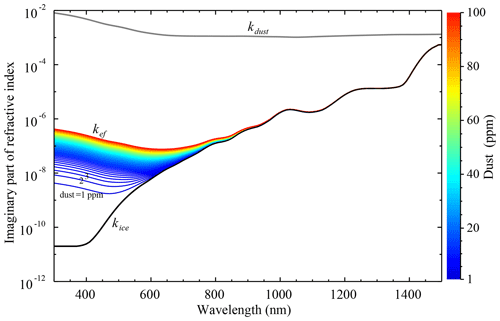Figure 3Imaginary part of the spectral complex refractive indices of ice (kice) and dust (kdust) (Warren and Brandt, 2008; Dang et al., 2015), with the imaginary part of the effective complex refractive indices (kef) as a function of wavelength, at dust mass concentrations (${\mathrm{C}}_{\mathrm{dust}}^{\ast }$) of 1–100 ppm (or µg g−1) in snow.

In all variations of the spatial distribution of dust particles in snow, the dust mass concentration was assumed to be constant, which means that the local dust fraction volume may differ. In an example of spherical ice grains with uniformly distributed dust, the dust fraction volume in an ice grain was determined as

$\begin{array}{}\text{(9)}& {\mathit{\delta }}_{\mathrm{dust}}^{\mathrm{0}}=\frac{{C}_{\mathrm{dust}}^{\ast }}{{C}_{\mathrm{v}}}\frac{{\mathit{\rho }}_{\mathrm{ice}}}{{\mathit{\rho }}_{\mathrm{dust}}},\end{array}$

where the ratio of ${C}_{\mathrm{dust}}^{\ast }/{C}_{\mathrm{v}}$ is the mass fraction of dust in the ice grain.

We considered two cases of nonuniform dust distributed in a spherical ice grain with radius ref. (1) We assumed that the same mass of dust is uniformly distributed in the central part of the ice grain (${r}_{\mathrm{ef}}^{\mathrm{dust}}<{r}_{\mathrm{c}}\le {r}_{\mathrm{ef}}$). (2) We assumed all of the dust was in the surface layer of the ice grain (${r}_{\mathrm{ef}}^{\mathrm{dust}}<{r}_{\mathrm{p}}\le {r}_{\mathrm{ef}}$) (Fig. 2). In both cases the local value of δdust increases as

$\begin{array}{}\text{(10)}& \begin{array}{rl}{\mathit{\delta }}_{\mathrm{dust}}& ={\mathit{\delta }}_{\mathrm{dust}}^{\mathrm{0}}/\mathit{\psi }\\ \mathit{\psi }& =\left\{\begin{array}{ll}{\stackrel{\mathrm{‾}}{{r}_{\mathrm{c}}}}^{\mathrm{3}}& \text{central pollution}\\ \mathrm{1}-{\left(\mathrm{1}-\stackrel{\mathrm{‾}}{{r}_{\mathrm{p}}}\right)}^{\mathrm{3}}& \text{peripheral pollution},\end{array}\right\\end{array}\end{array}$

where $\stackrel{\mathrm{‾}}{{r}_{\mathrm{c}}}={r}_{\mathrm{c}}/{r}_{\mathrm{ef}}$ and $\stackrel{\mathrm{‾}}{{r}_{\mathrm{p}}}={r}_{\mathrm{p}}/{r}_{\mathrm{ef}}$. $\stackrel{\mathrm{‾}}{{r}_{\mathrm{c}}}=\mathrm{1}$ and $\stackrel{\mathrm{‾}}{{r}_{\mathrm{p}}}=\mathrm{1}$ correspond to uniformly distributed dust when ψ=1 and ${\mathit{\delta }}_{\mathrm{dust}}={\mathit{\delta }}_{\mathrm{dust}}^{\mathrm{0}}$ and ψ<1 and ${\mathit{\delta }}_{\mathrm{dust}}>{\mathit{\delta }}_{\mathrm{dust}}^{\mathrm{0}}$ in other cases. Obviously, dust particles increase the imaginary part of the effective complex RIs in polluted ice grains (Fig. 3).

In summary, the mef of a spherical ice grain with uniformly and nonuniformly distributed can be calculated, according to Eqs. (8) to (10), then their corresponding absorption efficiencies can be obtained using classical Mie theory and core–shell Mie theory, respectively. The spectral snow albedo for IDM can be easily calculated using Eqs. (1) to (5).

## 2.3 Broadband snow albedo calculations

The spectral albedo (αλ) is integrated over the solar spectrum (λ=300–2500 nm) and weighted by incoming solar irradiance (Eλ) to calculate broadband snow albedo (Hadley and Kirchstetter, 2012):

$\begin{array}{}\text{(11)}& {\mathit{\alpha }}_{\mathrm{integrated}}=\frac{\int {\mathit{\alpha }}_{\mathit{\lambda }}{E}_{\mathit{\lambda }}\mathrm{d}\mathit{\lambda }}{\int {E}_{\mathit{\lambda }}\mathrm{d}\mathit{\lambda }}.\end{array}$

Following the study of Dang et al. (2017), the incoming solar irradiance we used is a typical surface solar spectrum at midlatitudes to high latitudes from January to March under the cloudy sky, calculated by the Santa Barbara DISORT Atmospheric Radiative Transfer (SBDART) model (Pu et al., 2019). The SBDART model is a widely used atmospheric radiation transfer model based on a collection of highly developed physical models, including the Discrete Ordinate Radiative Transfer module (Stamnes et al., 1988), low-resolution atmospheric transmission models, and Mie theory. The SBDART model can be used to compute radiative transfer at different heights and directions under both clear- and cloudy-sky conditions. Details on the SBDART model can be found in Ricchiazzi et al. (1998).

## 2.4 Dust concentration measurements

To estimate the effect of dust on snow albedo in real snowpack, we collected a comprehensive set of in situ dust concentration measurements during field campaigns to Inner Mongolia, China (IMC) (Wang et al., 2013); the Tibetan Plateau (TP) (Li et al., 2017, 2018, 2016, 2019; Niu et al., 2017; Qu et al., 2014; Zhang et al., 2017, 2018); Sapporo, Japan (SJ) (Kuchiki et al., 2015); the European Alps (EP) (Di Mauro et al., 2015; Lim et al., 2014); and North American mountains (NAM) (Painter et al., 2012; Reynolds et al., 2020). The field campaigns conducted in the TP can be further grouped into three regions, as in previous δ18O precipitation studies (Yao et al., 2013). These three distinct domains were associated with the Indian monsoon (Southern TP), westerlies (Northern TP), and transition (Central TP) (Yao et al., 2013). It is worth noting that we only considered regions with higher dust concentrations (>1 ppm), such that polar regions are not included in this study. Measurements of dust in the snow samples were generally obtained by weighing the filter before and after filtration using a microbalance.

3 Results

## 3.1 Impact on the imaginary part of the effective complex RIs

We evaluated the effect of dust on kef, including kice and kdust associated with absorption (Fig. 3). The kdust was in a narrow range (∼0.001–0.01) and gradually decreased with increasing wavelength in the ultraviolet (UV) and VIS regions and then remained stable in the NIR band. The kice varied by 8 orders of magnitude from the UV ($\sim {\mathrm{10}}^{-\mathrm{11}}$) to NIR ($\sim {\mathrm{10}}^{-\mathrm{3}}$) bands and increased with increasing wavelength, except at 1.03 µm where kice decreased slightly as a result of the presence of ice absorption features (Warren, 2019).

Figure 3 also shows the kef with dust mass concentrations ranging from 1 to 100 ppm and wavelengths of 300–1500 nm. The kef clearly varied depending on the wavelength and increased with dust mass concentrations. For a given dust mass concentration, kef decreased with wavelengths from UV to VIS and then increased from VIS to NIR. For example, the value of kef decreased from $\mathrm{4.26}×{\mathrm{10}}^{-\mathrm{8}}$ at 300 nm to $\mathrm{1.36}×{\mathrm{10}}^{-\mathrm{8}}$ at 500 nm and then rose to $\mathrm{1.73}×{\mathrm{10}}^{-\mathrm{6}}$ at 1000 nm at a dust concentration of 10 ppm. Moreover, the wavelength of the minimum of kef varied from ∼500 to ∼650 nm depending on the dust mass concentrations (1 to 100 ppm). Additionally, it is worth noting that kef was not sensitive to dust mass concentrations in wavelengths >1000 nm, which was generally consistent with kice, because the difference between kdust and kice is more than compensated for by the much larger difference in ice and dust concentration at those wavelengths. Conversely, kef showed significant differences relative to kice in the UV and VIS regions, with higher dust mass concentrations demonstrating larger differences. For example, kef was enhanced by a factor of 3, 21, and 205 at 500 nm relative to kice for dust mass concentrations of 1, 10, and 100 ppm, respectively.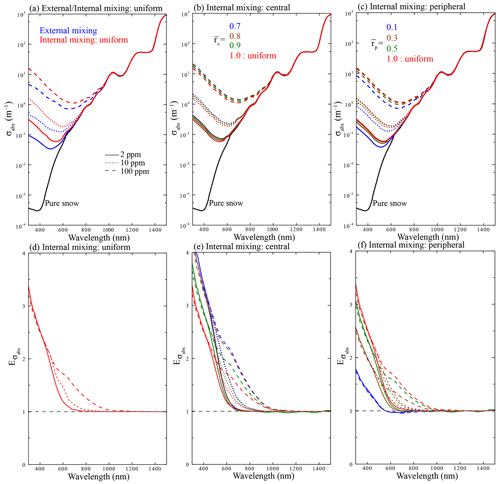Figure 4Snow absorption coefficients (σabs) for dust–snow (a) external and internal mixing (uniform), (b) internal mixing (central), and (c) internal mixing (peripheral), as a function of wavelength with different dust concentrations and $\stackrel{\mathrm{‾}}{{r}_{\mathrm{c}}}$ and $\stackrel{\mathrm{‾}}{{r}_{\mathrm{p}}}$. The corresponding enhancement (${\mathrm{E}}_{{\mathit{\sigma }}_{\mathrm{abs}}}$) caused by (d) internal mixing (uniform), (e) internal mixing (central), and (f) internal mixing (peripheral) relative to external mixing, is shown as a function of wavelength. The snow grain radius was assumed to be 200 µm.

## 3.2 Impact on spectral snow absorption coefficient and albedo

Dust in snow effectively enhances the snow absorption coefficient, but its effect on the snow asymmetry factor and extinction efficiency is negligible (He et al., 2019). Therefore, we mainly focused on the effects of EDM and three cases of IDM (uniform, central, and peripheral) on the snow absorption coefficient (σabs). Figure 4a displays the σabs for EDM and IDM (uniform) as a function of wavelength at different dust concentrations. We used a snow grain radius of 200 µm (Fig. 4), which is comparable to previous observations of seasonal snow at midlatitudes to high latitudes in winter (Shi et al., 2020; Wang et al., 2017). The results showed that EDM and IDM have distinct impacts on σabs in UV and VIS, but small effects at wavelengths >1000 nm, which is due to the optical properties of snow being affected by LAPs in UV and VIS and primarily affected by snow itself at wavelengths >1000 nm. Additionally, σabs increased with increased dust mass concentrations. For instance, σabs increased from 0.007 m−1 (pure snow) to 0.03, 0.14, and 1.37 m−1 at 500 nm with 2, 10, and 100 ppm of dust with EDM, respectively. For IDM (uniform), σabs increased to 0.06, 0.28, and 2.80 m−1 at 500 nm with 2, 10, and 100 ppm of dust, respectively, where corresponding enhancement factors of σabs (${E}_{{\mathit{\sigma }}_{\mathrm{abs}}}$, defined as the absorption coefficient of IDM divided by EDM) were 1.84, 2.00, and 2.05. Furthermore, the σabs for two cases of nonuniform dust distribution in a spherical ice grain (ref=200µm) depends on the wavelength, dust mass concentrations, $\stackrel{\mathrm{‾}}{{r}_{\mathrm{c}}}$, and $\stackrel{\mathrm{‾}}{{r}_{\mathrm{p}}}$ (Fig. 4b and c). We note that $\stackrel{\mathrm{‾}}{{r}_{\mathrm{c}}}$ and $\stackrel{\mathrm{‾}}{{r}_{\mathrm{p}}}$ values of 1 correspond to uniformly distributed dust, and the σabs increases and decreases (with the decrease in $\stackrel{\mathrm{‾}}{{r}_{\mathrm{c}}}$ and $\stackrel{\mathrm{‾}}{{r}_{\mathrm{p}}}$) for IDM (central) and IDM (peripheral), respectively. For example, σabs increased by 29 %, 32 %, and 33 % (500 nm) at dust mass concentrations of 2, 10, and 100 ppm, respectively, when $\stackrel{\mathrm{‾}}{{r}_{\mathrm{c}}}$ decreased from 1 to 0.7 for IDM (central). However, σabs decreased by 41 %, 44 %, and 44 % (500 nm) at dust mass concentrations of 2, 10, and 100 ppm, respectively, when $\stackrel{\mathrm{‾}}{{r}_{\mathrm{p}}}$decreased from 1 to 0.1 for IDM (peripheral). This indicates that the IDM (central) further enhanced snowpack light absorption compared with the IDM (uniform), while the IDM (peripheral) reduced snowpack light absorption with a corresponding σabs between the value of σabs for IDM (uniform) and EDM. Figure 4d–f quantitatively show the spectral snow absorption coefficient enhancement for IDM (${E}_{{\mathit{\sigma }}_{\mathrm{abs}}}$). The enhancement decreased sharply with increasing wavelengths and then reduced to 1.0 (i.e., no enhancement) at wavelengths longer than ∼1.0µm because of strong dust absorption and weak snow absorption at shorter wavelengths. Obviously, ${E}_{{\mathit{\sigma }}_{\mathrm{abs}}}$ was affected by dust mass concentration, $\stackrel{\mathrm{‾}}{{r}_{\mathrm{c}}}$, and $\stackrel{\mathrm{‾}}{{r}_{\mathrm{p}}}$, but ${E}_{{\mathit{\sigma }}_{\mathrm{abs}}}$ was insensitive to dust mass concentration at wavelengths <450 nm.

Figure 5a–c show the spectral snow albedo (αλ) for EDM and IDM; αλ was consistent with σabs, with the effects mainly present at wavelengths <1000 nm. Generally, αλ decreased with increased dust mass concentrations in UV and VIS, and IDM was shown to further trigger the reduction of αλ. For example, for EDM αλ was ∼0.97, 0.95, and 0.85 (at 500 nm) for dust concentrations of 2, 10, and 100 ppm, respectively, which was higher than the values for IDM (uniform) (∼0.96, 0.93, and 0.79, respectively). Compared with IDM (uniform), the αλ for IDM (central) decreased by 0.5 %, 1.1 %, and 3.5 % (at 500 nm) for dust concentrations of 2, 10, and 100 ppm, respectively, when $\stackrel{\mathrm{‾}}{{r}_{\mathrm{c}}}$ decreased from 1 to 0.7. αλ for IDM (peripheral) increased by 0.8 %, 1.9 %, and 6.2 % (at 500 nm) for the same dust concentrations, when $\stackrel{\mathrm{‾}}{{r}_{\mathrm{p}}}$ decreased from 1 to 0.1. Moreover, the wavelength of the maximum αλ value varied from ∼500 to ∼650 nm depending on the dust mass concentrations, which is consistent with changes of kef. Figure 5d–f show the ratio (${E}_{{\mathit{\alpha }}_{\mathit{\lambda }}}$) of snow spectral albedo for IDM to EDM where we observed that the ${E}_{{\mathit{\alpha }}_{\mathit{\lambda }}}$ increased with increasing wavelengths and dust concentrations and then became stable at 1.0. This is because IDM can enhance the light absorption of snowpack more effectively at shorter wavelengths and higher dust concentrations (Fig. 4). Additionally, the values of $\stackrel{\mathrm{‾}}{{r}_{\mathrm{c}}}$ and $\stackrel{\mathrm{‾}}{{r}_{\mathrm{p}}}$ also have non-negligible effects on ${E}_{{\mathit{\alpha }}_{\mathit{\lambda }}}$, which can be decreased and increased with decreasing $\stackrel{\mathrm{‾}}{{r}_{\mathrm{c}}}$ and $\stackrel{\mathrm{‾}}{{r}_{\mathrm{p}}}$, respectively.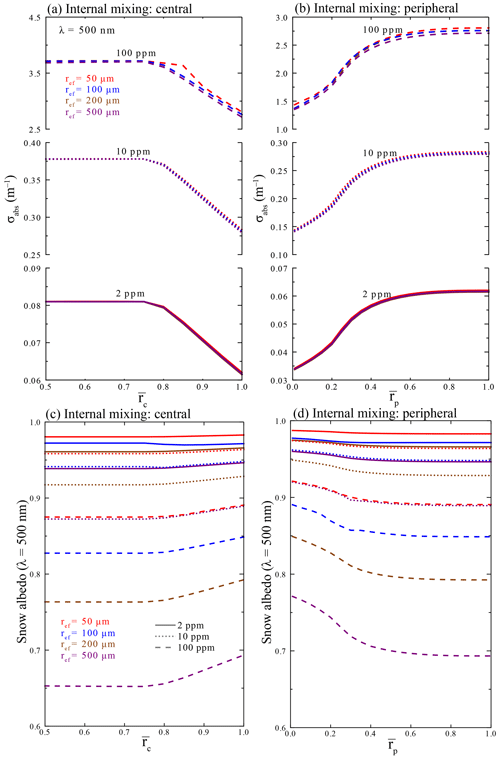Figure 6The snow absorption coefficient (σabs) at the 500 nm wavelength as a function of $\stackrel{\mathrm{‾}}{{r}_{\mathrm{c}}}$ and $\stackrel{\mathrm{‾}}{{r}_{\mathrm{p}}}$ for (a) internal mixing (central) and (b) internal mixing (peripheral) with different snow grain radii and dust mass concentrations. Panels (c) and (d) are the same as (a) and (b), but for snow albedo at the 500 nm wavelength.

We found that the optical properties of an ice grain containing uniformly distributed dust in its center, or concentric surface layer, can be affected by $\stackrel{\mathrm{‾}}{{r}_{\mathrm{c}}}$ or $\stackrel{\mathrm{‾}}{{r}_{\mathrm{p}}}$. To better understand this effect, Fig. 6a–b display the σabs at 500 nm as a function of $\stackrel{\mathrm{‾}}{{r}_{\mathrm{c}}}$ and $\stackrel{\mathrm{‾}}{{r}_{\mathrm{p}}}$ with different dust concentrations and ref. This demonstrates ref has negligible effects on σabs due to the geometric optical limits at ref≈50µm, which shows the universal (independent of ref) monotonic dependence of σabs on $\stackrel{\mathrm{‾}}{{r}_{\mathrm{c}}}$ and $\stackrel{\mathrm{‾}}{{r}_{\mathrm{p}}}$ for ice grains with ref≥50µm (Velesco et al., 1997). As a result, the spectral absorption coefficient of snow containing polydispersed ice grains can be obtained using our results for a monodispersed model. Interestingly, σabs did not depend on $\stackrel{\mathrm{‾}}{{r}_{\mathrm{c}}}$ when $\stackrel{\mathrm{‾}}{{r}_{\mathrm{c}}}<\mathrm{0.75}$ and decreased almost linearly at higher $\stackrel{\mathrm{‾}}{{r}_{\mathrm{c}}}$ values (Fig. 6a); this phenomenon can be explained by geometric optical effects (Mackowski et al., 1990). However, σabs was significantly affected by the dust mass concentration; for example, σabs at 500 nm was decreased by 28 %, 32 %, and 32 % from its maximum value (0.08, 0.38, and 3.71 m−1) for dust concentrations of 2, 10, and 100 ppm, respectively, when $\stackrel{\mathrm{‾}}{{r}_{\mathrm{c}}}$ increased from <0.75 to 1.0. The monotonic increase in σabs with the relative thickness of the polluted ice grain surface layer (i.e., $\stackrel{\mathrm{‾}}{{r}_{\mathrm{p}}}$) was also noteworthy (Fig. 6b). The core–shell Mie theory calculations for ice grains with a thin surface layer ($\stackrel{\mathrm{‾}}{{r}_{\mathrm{p}}}=\mathrm{0.01}$) gave almost the same σabs as that obtained for the EDM. As a result, the σabs increased rapidly with $\stackrel{\mathrm{‾}}{{r}_{\mathrm{p}}}<\mathrm{0.4}$ and then increased more slowly until $\stackrel{\mathrm{‾}}{{r}_{\mathrm{p}}}=\mathrm{1}$, which corresponds to IDM (uniform).

The αλ at 500 nm as a function of $\stackrel{\mathrm{‾}}{{r}_{\mathrm{c}}}$ and $\stackrel{\mathrm{‾}}{{r}_{\mathrm{p}}}$ with different dust concentrations and ref is illustrated in Fig. 6c and d. In general, αλ at 500 nm decreased with increasing dust mass concentration and ref; the effect of grain radius can be explained by the fact that the snow extinction coefficient is inversely proportional to ref, so that for a given amount of dust, the single-scattering albedo of the snow–dust mixture is smaller for large snow grains (Gardner and Sharp, 2010). For a given dust mass concentration and ref, the αλ at 500 nm increased from its minimum value with $\stackrel{\mathrm{‾}}{{r}_{\mathrm{c}}}<\mathrm{0.75}$ to the maximum value with $\stackrel{\mathrm{‾}}{{r}_{\mathrm{c}}}=\mathrm{1}$, corresponding to the findings of IDM (uniform). For example, at dust concentrations of 2, 10, and 100 ppm, and a fixed ref of 100 µm, the αλ at 500 nm increased by 0.1 %, 0.7 %, and 2.6 %, respectively, when $\stackrel{\mathrm{‾}}{{r}_{\mathrm{c}}}$ increased from <0.75 to 1. When the ref was fixed at 500 µm, the αλ at 500 nm increased by 0.8 %, 1.9 %, and 6.2 % at dust concentrations of 2, 10, and 100 ppm, respectively. Conversely, the αλ at 500 nm decreased from its maximum value when $\stackrel{\mathrm{‾}}{{r}_{\mathrm{p}}}=\mathrm{0.01}$ (similar to EDM) to the minimum value with $\stackrel{\mathrm{‾}}{{r}_{\mathrm{p}}}=\mathrm{1}$, corresponding to the case of IDM (uniform). For example, the αλ at 500 nm decreased by 0.6 %, 1.4 %, and 4.7 % when $\stackrel{\mathrm{‾}}{{r}_{\mathrm{p}}}$ increased from 0.01 to 1 for dust concentrations of 2, 10, and 100 ppm, respectively, and a fixed ref of 100 µm, whereas for a ref of 500 µm, αλ decreased by 1.4 % (2 ppm dust), 3.3 % (10 ppm), and 10.1 % (100 ppm). These results indicate that dust mass concentrations and ref can amplify the influence of $\stackrel{\mathrm{‾}}{{r}_{\mathrm{c}}}$ or $\stackrel{\mathrm{‾}}{{r}_{\mathrm{p}}}$ on snow albedo. Moreover, the effect of dust mass concentration on snow albedo is similar to ref. For example, dust mass concentrations of 10 and 100 ppm and ref of 100 and 50 µm gave similar αλ at 500 nm to dust mass concentrations of 2 and 10 ppm and ref of 500 µm. According to this result, spectral albedo measurements at a single wavelength are insufficient to obtain the mass fraction of dust in snow cover because the same effect can also be explained by a combination of different ice grain sizes and a nonuniform distribution of dust inside the grains. It means that additional information is needed to determine accurate dust mass concentrations. This may be a set of measurements at various wavelengths in the VIS and NIR spectral ranges.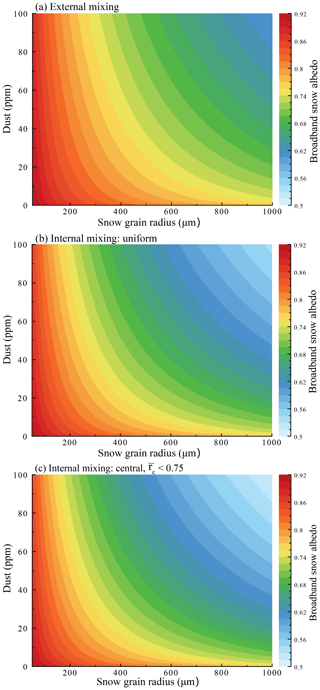Figure 7Broadband snow albedo (αintegrated) variations affected by different dust mass concentrations and snow grain radii for (a) external mixing, (b) internal mixing (uniform), and (c) internal mixing (central, $\stackrel{\mathrm{‾}}{{r}_{\mathrm{c}}}<\mathrm{0.75}$).

## 3.3 Effects on broadband snow albedo

Compared with the spectral optical properties, broadband results can provide more general knowledge for the relevant research community. Figure 7 shows the spectrally weighted αλ (αintegrated) over 300–2500 nm of a typical surface solar spectrum at midlatitudes to high latitudes, which is comparable with previous studies (Dang et al., 2017; Wang et al., 2017). Because the results of IDM (peripheral) effects on snow albedo fell between results from EDM and IDM (central), we do not consider the case of IDM (peripheral) in the following discussion. Instead, we focus on the effects of dust mass concentration and ref on broadband snow albedo for EDM and IDM (uniform, central). Similar to αλ, αintegrated generally decreased with increasing dust mass concentrations and ref such that αintegrated declined more for internal mixing than external mixing. αintegrated showed ranges of 0.60–0.92, 0.54–0.92, and 0.51–0.92 for EDM, IDM (uniform), and IDM (central, $\stackrel{\mathrm{‾}}{{r}_{\mathrm{c}}}<\mathrm{0.75}$), respectively, with dust mass concentrations of 0–100 ppm and ref of 50–1000 µm. For a given dust mass concentration and ref, αintegrated for IDM (uniform) was smaller than EDM, which is due to higher light absorption in the UV and VIS bands for IDM (uniform) relative to EDM (Fig. 4a). While αintegrated for IDM (uniform) was larger compared with IDM (central, $\stackrel{\mathrm{‾}}{{r}_{\mathrm{c}}}<\mathrm{0.75}$), this can be attributed to the fact that radiation is focused near the center of an ice grain with IDM (central) (Ackerman and Toon, 1981; Bohren, 1986), enabling further absorptions from inclusions near the center of a grain due to the lensing effect (Mackowski et al., 1990). For example, αintegrated (dust concentration of 20 ppm, ref of 500 µm) was 0.73 for IDM (uniform), less than 0.76 for EDM, but higher than (central, $\stackrel{\mathrm{‾}}{{r}_{\mathrm{c}}}<\mathrm{0.75}$) 0.72 for IDM.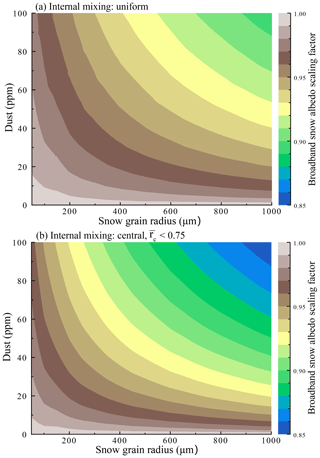Figure 8Variations in the broadband snow albedo scaling factor (${E}_{{\mathit{\alpha }}_{\mathrm{integrated}}}$, ratio of αintegrated for IDM to EDM) due to different dust mass concentrations and snow grain radii for (a) internal mixing (uniform) and (b) internal mixing (central, $\stackrel{\mathrm{‾}}{{r}_{\mathrm{c}}}<\mathrm{0.75}$).

To quantify the effects of IDM on broadband snow albedo relative to EDM, we defined a broadband snow albedo scaling factor (${E}_{{\mathit{\alpha }}_{\mathrm{integrated}}}$), which refers to the ratio of αintegrated of IDM to EDM. Generally, for dust mass concentrations from 0 to 100 ppm and ref of 50–1000 µm, ${E}_{{\mathit{\alpha }}_{\mathrm{integrated}}}$ varied from 0.89 to ∼1.00 for IDM (uniform) (Fig. 8a) and from 0.85 to ∼1.00 for IDM (central, $\stackrel{\mathrm{‾}}{{r}_{\mathrm{c}}}<\mathrm{0.75}$) (Fig. 8b). ${E}_{{\mathit{\alpha }}_{\mathrm{integrated}}}$ decreased significantly with increasing dust mass concentration and ref. In addition, ${E}_{{\mathit{\alpha }}_{\mathrm{integrated}}}$ for IDM (central, $\stackrel{\mathrm{‾}}{{r}_{\mathrm{c}}}<\mathrm{0.75}$) was smaller than IDM (uniform). These results have implications for the effects of IDM in real environments. For example, IMC has typical dust concentrations of ∼10 ppm (Wang et al., 2013), so ${E}_{{\mathit{\alpha }}_{\mathrm{integrated}}}$ (ref of 50–1000 µm) was 0.96–0.99 and 0.95–0.99 for IDM (uniform) and IDM (central, $\stackrel{\mathrm{‾}}{{r}_{\mathrm{c}}}<\mathrm{0.75}$), respectively. In contrast, dust concentrations are typically ∼100 ppm in the TP (Ming et al., 2016; Li et al., 2017, 2018), so ${E}_{{\mathit{\alpha }}_{\mathrm{integrated}}}$ ranged from 0.89 to 0.98 and 0.85 to 0.96 for IDM (uniform) and IDM (central, $\stackrel{\mathrm{‾}}{{r}_{\mathrm{c}}}<\mathrm{0.75}$), respectively. The results show that IDM (uniform) and IDM (central, $\stackrel{\mathrm{‾}}{{r}_{\mathrm{c}}}<\mathrm{0.75}$) reduced broadband snow albedo by ∼2.5 % and ∼3.0 %, respectively, in clean snow and ∼6.5 % and ∼9.5 %, respectively, in polluted snow relative to EDM. Moreover, the sensitivity of ${E}_{{\mathit{\alpha }}_{\mathrm{integrated}}}$ to mineral dust decreased with increasing dust concentrations. For example, the difference in ${E}_{{\mathit{\alpha }}_{\mathrm{integrated}}}$ (ref of 500 µm) between dust concentrations of 10 and 20 ppm was 0.011 and 0.015 for IDM (uniform) and IDM (central, $\stackrel{\mathrm{‾}}{{r}_{\mathrm{c}}}<\mathrm{0.75}$), respectively, while the corresponding differences between dust concentrations of 90 and 100 ppm were only 0.004 and 0.005. These results provide a convenient method to calculate the albedo of IDM when the albedo of EDM has been obtained for a given dust mass concentration and ref.

## 3.4 Uncertainties

Although we calculated the imaginary RI values of dust using previous studies (Sect. 2.2), there are still large variations which strongly depend on dust composition (e.g., hematite/iron content) (Balkanski et al., 2007; Wagner et al., 2012). To roughly account for this, we estimated the influence of chosen imaginary RI values on spectrally weighted snow albedo (${E}_{{\mathit{\alpha }}_{\mathrm{integrated}}}$) by increasing and decreasing the calculated imaginary RI values by 50 %. These changes in imaginary RIs are plausible because they are consistent with other studies (McConnell et al., 2010; Wagner et al., 2012). The results showed that ${E}_{{\mathit{\alpha }}_{\mathrm{integrated}}}$ uncertainties attributed to the imaginary RIs of dust were ±3.9 % and ±5.2 % for IDM (uniform) and IDM (central, $\stackrel{\mathrm{‾}}{{r}_{\mathrm{c}}}<\mathrm{0.75}$), respectively.

In contrast, observations have displayed large variations in the size distribution of dust in the atmosphere and snow, and this variation is strongly affected by the dust source and transport (Mahowald et al., 2014; Shao et al., 2011). In our standard simulation, we assumed a log-normal dust size distribution with a geometric mean diameter of 0.65 µm and a standard deviation of 2.0 (equivalent to an effective radius of 1.1 µm), which is typical for dust transported over a long range (Formenti et al., 2011; Maring et al., 2003); closer sources of dust tend to be larger (Kok, 2011). Therefore, we investigated the effects of dust particle size on our results by assuming another two log-normal size distributions with effective radii of 2.5 and 5.0 µm, which were within the observed size ranges in the atmosphere and snow and comparable with previously analyzed dust particle sizes (Maring et al., 2003; Shao and Mao, 2016; Zhang et al., 2003). The results showed that the uncertainty of ${E}_{{\mathit{\alpha }}_{\mathrm{integrated}}}$ attributed to dust diameter was ±6.1 % for both IDM (uniform) and IDM (central, $\stackrel{\mathrm{‾}}{{r}_{\mathrm{c}}}<\mathrm{0.75}$), but it should be emphasized that this uncertainty estimate accounts only for the impact of dust size on snow albedo in the case of external mixing, since the impact on albedo due to dust size cannot be evaluated when using the effective medium approximation. Overall, the total uncertainty of ${E}_{{\mathit{\alpha }}_{\mathrm{integrated}}}$ from variations in imaginary RIs and dust diameter was ±11.0 % and ±11.2 % for IDM (uniform) and IDM (central, $\stackrel{\mathrm{‾}}{{r}_{\mathrm{c}}}<\mathrm{0.75}$), respectively, with dust mass concentrations of 0–100 ppm and ref of 50–1000 µm. Specifically, the uncertainty increased with increasing ref and dust concentrations.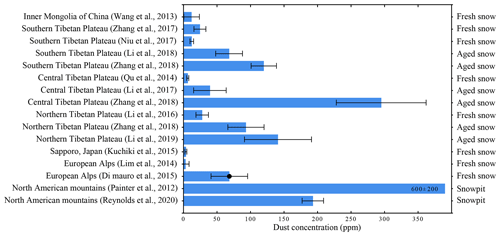Figure 9In situ measurements of dust concentrations in snow (fresh snow, aged snow, and snow pit from field sampling in different regions of the Northern Hemisphere). The solid black circle represents snow samples that were collected days after a significant dust transport event.

## 3.5 Measurement-based estimate of the effects of dust on snow albedo

Finally, widespread dust concentrations in snow across the Northern Hemisphere were obtained to assess the effects of dust on snow albedo in real snowpack. Figure 9 shows measured dust concentrations in snow in different regions; dust concentrations spanned a broad range of values because of spatial and temporal variations in emissions, transportation, and deposition among the different regions. Dust concentrations widely varied from ∼3 to ∼600 ppm, with the highest concentration in NAM and lowest in the EP (Di Mauro et al., 2015; Lim et al., 2014; Painter et al., 2012; Reynolds et al., 2020). However, snow samples collected in the days after a significant dust transport event showed that dust concentrations in snow can be up to ∼70 ppm in the EP (Di Mauro et al., 2015). Additionally, the average dust concentrations in fresh snow were 18, 6, and 28 ppm in the southern TP, central TP, and northern TP, respectively, similar to the IMC (12 ppm) (Wang et al., 2013). However, dust concentrations in aged snow (120, 300, and 140 ppm) were 1 to 2 orders of magnitude higher than in fresh snow, indicating the important correlation between snow type and dust concentration (Zhang et al., 2017, 2018).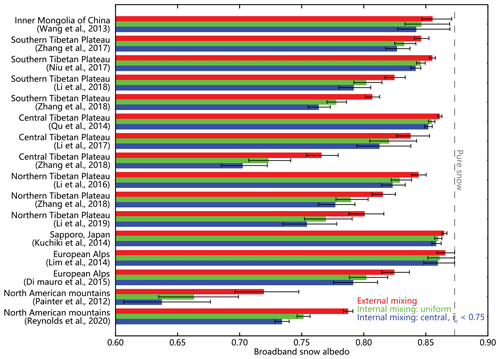Figure 10Calculated broadband snow albedo based on dust concentration measurements in different areas for dust–snow external mixing, internal mixing (uniform), and internal mixing (central, $\stackrel{\mathrm{‾}}{{r}_{\mathrm{c}}}<\mathrm{0.75}$). The dashed line represents broadband albedo of pure snow, and the snow grain radius was assumed to be 200 µm.

We calculated the broadband snow albedo for EDM, IDM (uniform), and IDM (central, $\stackrel{\mathrm{‾}}{{r}_{\mathrm{c}}}<\mathrm{0.75}$) based on the measured dust concentrations (Fig. 10). The results showed that broadband snow albedo decreased by 0.8 %, 1.4 %, and 1.6 % in the EP for EDM, IDM (uniform), and IDM (central, $\stackrel{\mathrm{‾}}{{r}_{\mathrm{c}}}<\mathrm{0.75}$), respectively, which was similar to SJ. However, the broadband snow albedo decreased by up to 5.6 %, 8.1 %, and 9.4 % in the EP after a significant dust transport event, indicating strong snow albedo reduction during these events. In addition, broadband snow albedo was reduced by 2.0 %, 3.1 %, and 3.6 % in IMC for EDM, IDM (uniform), and IDM (central, $\stackrel{\mathrm{‾}}{{r}_{\mathrm{c}}}<\mathrm{0.75}$), respectively. Similar results were also found for the southern TP, central TP, and northern TP where the broadband snow albedo for fresh snow was reduced by 2.5 %, 1.4 %, and 3.3 %, respectively, for EDM; 3.9 %, 2.1 %, and 5.1 % for IDM (uniform); and 4.5 %, 2.4 %, and 5.7 % for IDM (central, $\stackrel{\mathrm{‾}}{{r}_{\mathrm{c}}}<\mathrm{0.75}$). However, the broadband snow albedo was more significantly reduced for aged snow: up to 6.0 %, 8.1 %, and 7.5 % for EDM; 9.5 %, 11.6 %, and 10.5 % for IDM (uniform); and 10.9 %, 13.2 %, and 12.3 % for IDM (central, $\stackrel{\mathrm{‾}}{{r}_{\mathrm{c}}}<\mathrm{0.75}$) in the southern, central, and northern TP, respectively. This indicates that the effects of dust on snow albedo showed stronger reductions during snowmelt periods. It is worth noting that the effects of dust on snow albedo for aged snow could be underestimated due to the larger snow grain effective radius (ref) than fresh snow. Moreover, the largest broadband snow albedo reductions were found in NAM with ranges of 9.8 %–17.6 %, 13.9 %–24.1 %, and 15.9 %–27.0 % for EDM, IDM (uniform), and IDM (central, $\stackrel{\mathrm{‾}}{{r}_{\mathrm{c}}}<\mathrm{0.75}$), respectively. These results suggest that the effects of external or internal dust–snow mixing on snow albedo are particularly significant for the TP and NAM regions, with stronger reductions in albedo. Therefore, these results can have significant impacts on both local hydrological cycles and regional climate change (Oaida et al., 2015; Xie et al., 2018).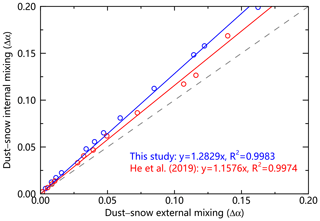Figure 11Comparisons of snow albedo reduction (Δα) under the cloudy sky caused by dust–snow uniform internal mixing (y axis) and external mixing (x axis) for this study (blue) and He et al. (2019) (red).

4 Discussion

In this study, the application of the effective medium approximation greatly simplifies the complexity of snow radiation transfer calculation for dust–snow internal mixing, and the effect of nonuniform distribution of dust in snow grains on snowpack optical properties are explicitly quantified for the first time. However, it is worth noting that this method has its limitation when applying to large particles (e.g., dust) in snow (Bohren, 1986; Flanner et al., 2012), which can create some errors for the albedo calculation of dust–snow internal mixing. To verify the credibility of our results, we carefully make a comparison with the more rigorous calculations found in He et al. (2019), which used the geometric-optics surface-wave approach (GOS) to consider the impact of dust–snow uniform internal mixing on snow albedo reduction. As shown in Fig. 11, the results show that the enhancement ratio of snow albedo reduction (1.28) due to dust–snow internal mixing (relative to external mixing) is slightly higher than the value (1.16) reported by He et al. (2019), and this deviation is comparable to that caused by snow nonsphericity (He et al., 2019). Therefore, we indicated that the effective medium approximation used in this study is reasonable and reliable.

Over the past few decades, the effects of dust in snow on reductions in albedo have been widely demonstrated (Skiles et al., 2018; Zhang et al., 2018). However, the magnitude of these effects has only been studied in a few regions, and uncertainties still remain. Our study indicates that the albedo of dust-contaminated snowpack can be affected by the dust–ice mixing state. In particular, IDM enhanced light absorption and reduced snow albedo more significantly compared with EDM. For example, in IMC and the TP, IDM reduced snow albedo by ∼5 % relative to EDM at a typical dust mass concentration of 20 ppm and a snow grain radius of 500 µm. This exceeds the contribution of BC to snow light absorption over most areas of North America and the Arctic (Dang et al., 2017). In addition, the effects of IDM on snow albedo were amplified by higher dust mass concentrations and larger snow grain sizes. We therefore strongly suggest that IDM must be considered in future climate models, particularly to more accurately evaluate the climate in areas where snowpack is heavily contaminated with dust and is experiencing melting.

The mixed state between dust and snow gradually progresses from partial external mixing to wholly internally mixed. Therefore, assuming a completely external mixing of dust and snow grains will underestimate the effects of dust on snow albedo and radiative forcing in numerical models (e.g., Dang et al., 2015; Nagorski et al., 2019). Similarly, assuming completely internal mixing of dust and snow grains will overestimate the effects of dust (e.g., He et al., 2019; Liou et al., 2014). Zhao et al. (2014) underestimated the effects of dust by treating wet-deposited dust as externally mixed with snow grains. In future studies, we recommend the actual ratio between external and internal mixing for dust in snow be examined with an environmental scanning electron microscope equipped with a cold stage.

5 Conclusions

In this study, the effects of dust particles on absorption coefficients and snow albedo were theoretically analyzed by combining asymptotic radiative transfer theory and (core–shell) Mie theory. We initially considered external mixing – when dust is present between ice grains – and variations in internal mixing of dust within ice grains. We found that snow spectral absorption coefficients of IDM were larger than EDM across UV to NIR wavelengths but were negligible at wavelengths >1000 nm. The absorption enhancement (relative to EDM) was wavelength-dependent and increased with increased dust concentrations.

Compared with a uniform distribution of dust particles in ice grains, our calculations showed that nonuniformly distributed dust particles may lead to significantly different snow spectral absorption coefficients in the VIS band. Snow spectral absorption coefficients were further increased when all of the dust was positioned in the central part of ice grains, while the maximum absorption coefficient was found when the radius of a dust-polluted core was <75 % of the ice grain radius. In contrast, snow spectral absorption coefficients decreased when all of the dust was positioned in the surface layer of ice grains, and the minimum absorption coefficient was observed in the thin surface layer of dust-polluted ice grains, which was similar for EDM. As a result, broadband snow albedo decreased by up to 21 %, 30 %, and 33 % for EDM, IDM (uniform), and IDM (central, $\stackrel{\mathrm{‾}}{{r}_{\mathrm{c}}}<\mathrm{0.75}$), respectively, at dust concentrations of 100 ppm and ref of 1000 µm.

Based on comprehensive field measurements across the Northern Hemisphere, the effect of dust on snow albedo in real snowpack was evaluated by assuming external and internal dust–snow mixing. The largest reductions in broadband snow albedo were in NAM because that region had the highest average dust concentrations; IDM (uniform) and IDM (central, $\stackrel{\mathrm{‾}}{{r}_{\mathrm{c}}}<\mathrm{0.75}$) further decreased snow albedo by 4.6 %–7.8 % and 6.8 %–11.4 %, respectively, compared with EDM. This implies an important influence of internal dust–snow mixing in NAM.

Code availability

The code of (core–shell) Mie theory used in this study can be found at http://eodg.atm.ox.ac.uk/MIE/mie_single.html (last access: 20 April 2021, Earth Observation Data Group, 2021) and http://gwest.gats-inc.com/software/bhcoat.pro (last access: 20 April 2021, Hervig, 2021).

Data availability

The data used for this study are available via a Zenodo archive, which can be found at https://doi.org/10.5281/zenodo.4704673 (Shi, 2021).

Author contributions

XW designed the study and evolved the overarching research goals and aims. TS wrote the first draft with contributions from all co-authors. TS and CJ applied formal techniques such as statistical, mathematical, and computational to analyze study data. YC and XX collected the dust measurements across the Northern Hemisphere. YZ and WP provided the majority of the methodology and software. QC and XZ provided technical guidance. All authors contributed to the improvement of results and revised the final paper.

Competing interests

The authors declare that they have no conflict of interest.

Special issue statement

Financial support

This research has been supported by the National Science Fund for Distinguished Young Scholars (grant no. 42025102), the National Key Research and Development Program of China (grant no. 2019YFA0606801), the National Natural Science Foundation of China (grant nos. 41975157, 41775144, and 42075061), and the Project of Science and Technology Plan of Sichuan (grant no. 2019YJ0363).

Review statement

This paper was edited by Stelios Kazadzis and reviewed by four anonymous referees.

References

Ackerman, T. P. and Toon, O. B.: Absorption of Visible Radiation in Atmosphere Containing Mixtures of Absorbing and Non-Absorbing Particles, Appl. Optics., 20, 3661–3668, https://doi.org/10.1364/Ao.20.003661, 1981.

Aoki, T., Aoki, T., Fukabori, M., Hachikubo, A., Tachibana, Y., and Nishio, F.: Effects of snow physical parameters on spectral albedo and bidirectional reflectance of snow surface, J. Geophys. Res.-Atmos., 105, 10219–10236, https://doi.org/10.1029/1999jd901122, 2000.

Balkanski, Y., Schulz, M., Claquin, T., and Guibert, S.: Reevaluation of Mineral aerosol radiative forcings suggests a better agreement with satellite and AERONET data, Atmos. Chem. Phys., 7, 81–95, https://doi.org/10.5194/acp-7-81-2007, 2007.

Bohren, C. F.: Applicability of Effective-Medium Theories to Problems of Scattering and Absorption by Nonhomogeneous Atmospheric Particles, J. Atmos. Sci., 43, 468–475, https://doi.org/10.1175/1520-0469(1986)043<0468:Aoemtt>2.0.Co;2, 1986.

Casey, K. A., Kaspari, S. D., Skiles, S. M., Kreutz, K., and Handley, M. J.: The spectral and chemical measurement of pollutants on snow near South Pole, Antarctica, J. Geophys. Res.-Atmos., 122, 6592–6610, https://doi.org/10.1002/2016jd026418, 2017.

Creamean, J. M., Suski, K. J., Rosenfeld, D., Cazorla, A., DeMott, P. J., Sullivan, R. C., White, A. B., Ralph, F. M., Minnis, P., Comstock, J. M., Tomlinson, J. M., and Prather, K. A.: Dust and Biological Aerosols from the Sahara and Asia Influence Precipitation in the Western U.S., Science, 339, 1572–1578, https://doi.org/10.1126/science.1227279, 2013.

Dang, C., Brandt, R. E., and Warren, S. G.: Parameterizations for narrowband and broadband albedo of pure snow and snow containing mineral dust and black carbon, J. Geophys. Res.-Atmos., 120, 5446–5468, https://doi.org/10.1002/2014JD022646, 2015.

Dang, C., Fu, Q., and Warren, S. G.: Effect of Snow Grain Shape on Snow Albedo, J. Atmos. Sci., 73, 3573–3583, https://doi.org/10.1175/JAS-D-15-0276.1, 2016.

Dang, C., Warren, S. G., Fu, Q., Doherty, S. J., Sturm, M., and Su, J.: Measurements of light-absorbing particles in snow across the Arctic, North America, and China: Effects on surface albedo, J. Geophys. Res.-Atmos., 122, 10149–10168, https://doi.org/10.1002/2017jd027070, 2017.

Di Mauro, B., Fava, F., Ferrero, L., Garzonio, R., Baccolo, G., Delmonte, B., and Colombo, R.: Mineral dust impact on snow radiative properties in the European Alps combining ground, UAV, and satellite observations, J. Geophys. Res.-Atmos., 120, 6080–6097, https://doi.org/10.1002/2015jd023287, 2015.

Di Mauro, B., Garzonio, R., Baccolo, G., Franzetti, A., Pittino, F., Leoni, B., Remias, D., Colombo, R., and Rossini, M.: Glacier algae foster ice-albedo feedback in the European Alps, Sci. Rep.-Uk, 10, 4739, https://doi.org/10.1038/s41598-020-61762-0, 2020.

Dombrovsky, L. A. and Baillis, D.: Thermal radiation in disperse systems: an engineering approach, Begell House, New York, 2010.

Dombrovsky, L. A. and Kokhanovsky, A. A.: Light absorption by polluted snow cover: Internal versus external mixture of soot, J. Quant. Spectrosc. Ra., 242, 106799, https://doi.org/10.1016/j.jqsrt.2019.106799, 2020.

Earth Observation Data Group: mie_single.pro, available at: http://eodg.atm.ox.ac.uk/MIE/mie_single.html, last access: 20 April 2021.

Flanner, M. G., Zender, C. S., Randerson, J. T., and Rasch, P. J.: Present-day climate forcing and response from black carbon in snow, J. Geophys. Res., 112, D11202, https://doi.org/10.1029/2006jd008003, 2007.

Flanner, M. G., Shell, K. M., Barlage, M., Perovich, D. K., and Tschudi, M. A.: Radiative forcing and albedo feedback from the Northern Hemisphere cryosphere between 1979 and 2008, Nat. Geosci., 4, 151–155, https://doi.org/10.1038/ngeo1062, 2011.

Flanner, M. G., Liu, X., Zhou, C., Penner, J. E., and Jiao, C.: Enhanced solar energy absorption by internally-mixed black carbon in snow grains, Atmos. Chem. Phys., 12, 4699–4721, https://doi.org/10.5194/acp-12-4699-2012, 2012.

Formenti, P., Schütz, L., Balkanski, Y., Desboeufs, K., Ebert, M., Kandler, K., Petzold, A., Scheuvens, D., Weinbruch, S., and Zhang, D.: Recent progress in understanding physical and chemical properties of African and Asian mineral dust, Atmos. Chem. Phys., 11, 8231–8256, https://doi.org/10.5194/acp-11-8231-2011, 2011.

France, J. L., Reay, H. J., King, M. D., Voisin, D., Jacobi, H. W., Domine, F., Beine, H., Anastasio, C., MacArthur, A., and Lee-Taylor, J.: Hydroxyl radical and NOx production rates, black carbon concentrations and light-absorbing impurities in snow from field measurements of light penetration and nadir reflectivity of onshore and offshore coastal Alaskan snow, J. Geophys. Res.-Atmos., 117, D00R12, https://doi.org/10.1029/2011jd016639, 2012.

Gabbi, J., Huss, M., Bauder, A., Cao, F., and Schwikowski, M.: The impact of Saharan dust and black carbon on albedo and long-term mass balance of an Alpine glacier, The Cryosphere, 9, 1385–1400, https://doi.org/10.5194/tc-9-1385-2015, 2015.

Gardner, A. S. and Sharp, M. J.: A review of snow and ice albedo and the development of a new physically based broadband albedo parameterization, J. Geophys. Res., 115, F01009, https://doi.org/10.1029/2009jf001444, 2010.

Grenfell, T. C., Light, B., and Sturm, M.: Spatial distribution and radiative effects of soot in the snow and sea ice during the SHEBA experiment, J. Geophys. Res.-Oceans, 107, 8032, https://doi.org/10.1029/2000jc000414, 2002.

Hadley, O. L. and Kirchstetter, T. W.: Black-carbon reduction of snow albedo, Nat. Clim. Change, 2, 437–440, https://doi.org/10.1038/nclimate1433, 2012.

Hansen, J. and Nazarenko, L.: Soot climate forcing via snow and ice albedos, P. Natl. Acad. Sci. USA, 101, 423–428, https://doi.org/10.1073/pnas.2237157100, 2004.

He, C., Flanner, M. G., Chen, F., Barlage, M., Liou, K.-N., Kang, S., Ming, J., and Qian, Y.: Black carbon-induced snow albedo reduction over the Tibetan Plateau: uncertainties from snow grain shape and aerosol–snow mixing state based on an updated SNICAR model, Atmos. Chem. Phys., 18, 11507–11527, https://doi.org/10.5194/acp-18-11507-2018, 2018.

He, C., Liou, K.-N., Takano, Y., Chen, F., and Barlage, M.: Enhanced Snow Absorption and Albedo Reduction by Dust-Snow Internal Mixing: Modeling and Parameterization, J. Adv. Model. Earth. Sy., 11, 3755–3776, https://doi.org/10.1029/2019ms001737, 2019.

Hervig, M.: bhcoat.pro, available at: http://gwest.gats-inc.com/software/bhcoat.pro, last access: 20 April 2021.

Horhold, M. W., Laepple, T., Freitag, J., Bigler, M., Fischer, H., and Kipfstuhl, S.: On the impact of impurities on the densification of polar firn, Earth Planet. Sc. Lett., 325, 93–99, https://doi.org/10.1016/j.epsl.2011.12.022, 2012.

Huang, J. P., Wang, T. H., Wang, W. C., Li, Z. Q., and Yan, H. R.: Climate effects of dust aerosols over East Asian arid and semiarid regions, J. Geophys. Res.-Atmos., 119, 11398–11416, https://doi.org/10.1002/2014JD021796, 2014.

Jacobson, M. Z.: Climate response of fossil fuel and biofuel soot, accounting for soot's feedback to snow and sea ice albedo and emissivity, J. Geophys. Res.-Atmos., 109, D21201, https://doi.org/10.1029/2004jd004945, 2004.

Kaspari, S., Skiles, S. M., Delaney, I., Dixon, D., and Painter, T. H.: Accelerated glacier melt on Snow Dome, Mount Olympus, Washington, USA, due to deposition of black carbon and mineral dust from wildfire, J. Geophys. Res.-Atmos., 120, 2793–2807, https://doi.org/10.1002/2014jd022676, 2015.

Kok, J. F.: A scaling theory for the size distribution of emitted dust aerosols suggests climate models underestimate the size of the global dust cycle, P. Natl. Acad. Sci. USA, 108, 1016–1021, https://doi.org/10.1073/pnas.1014798108, 2011.

Kokhanovsky, A.: Spectral reflectance of solar light from dirty snow: a simple theoretical model and its validation, The Cryosphere, 7, 1325–1331, https://doi.org/10.5194/tc-7-1325-2013, 2013.

Kokhanovsky, A. A. and Zege, E. P.: Scattering optics of snow, Appl. Optics, 43, 1589–1602, https://doi.org/10.1364/AO.43.001589, 2004.

Koledintseva, M. Y., DuBroff, R. E., and Schwartz, R. W.: Maxwell Garnett Rule for Dielectric Mixtures with Statistically Distributed Orientations of Inclusions, Prog. Electromagn. Res., 99, 131–148, https://doi.org/10.2528/PIER09091605, 2009.

Kuchiki, K., Aoki, T., Niwano, M., Matoba, S., Kodama, Y., and Adachi, K.: Elemental carbon, organic carbon, and dust concentrations in snow measured with thermal optical and gravimetric methods: Variations during the 2007–2013 winters at Sapporo, Japan, J. Geophys. Res.-Atmos., 120, 868–882, https://doi.org/10.1002/2014JD022144, 2015.

Li, X. F., Kang, S. C., He, X. B., Qu, B., Tripathee, L., Jing, Z. F., Paudyal, R., Li, Y., Zhang, Y. L., Yan, F. P., Li, G., and Li, C. L.: Light-absorbing impurities accelerate glacier melt in the Central Tibetan Plateau, Sci. Total Environ., 587, 482–490, https://doi.org/10.1016/j.scitotenv.2017.02.169, 2017.

Li, X. F., Kang, S. C., Zhang, G. S., Qu, B., Tripathee, L., Paudyal, R., Jing, Z. F., Zhang, Y. L., Yan, F. P., Li, G., Cui, X. Q., Xu, R., Hu, Z. F., and Li, C. L.: Light-absorbing impurities in a southern Tibetan Plateau glacier: Variations and potential impact on snow albedo and radiative forcing, Atmos. Res., 200, 77–87, https://doi.org/10.1016/j.atmosres.2017.10.002, 2018.

Li, Y., Chen, J., Kang, S., Li, C., Qu, B., Tripathee, L., Yan, F., Zhang, Y., Guo, J., Gul, C., and Qin, X.: Impacts of black carbon and mineral dust on radiative forcing and glacier melting during summer in the Qilian Mountains, northeastern Tibetan Plateau, The Cryosphere Discuss. [preprint], https://doi.org/10.5194/tc-2016-32, 2016.

Li, Y., Kang, S., Chen, J., Hu, Z., Wang, K., Paudyal, R., Liu, J., Wang, X., Qin, X., and Sillanpää, M.: Black carbon in a glacier and snow cover on the northeastern Tibetan Plateau: Concentrations, radiative forcing and potential source from local topsoil, Sci. Total Environ., 686, 1030–1038, https://doi.org/10.1016/j.scitotenv.2019.05.469, 2019.

Libois, Q., Picard, G., France, J. L., Arnaud, L., Dumont, M., Carmagnola, C. M., and King, M. D.: Influence of grain shape on light penetration in snow, The Cryosphere, 7, 1803–1818, https://doi.org/10.5194/tc-7-1803-2013, 2013.

Lim, S., Faïn, X., Zanatta, M., Cozic, J., Jaffrezo, J.-L., Ginot, P., and Laj, P.: Refractory black carbon mass concentrations in snow and ice: method evaluation and inter-comparison with elemental carbon measurement, Atmos. Meas. Tech., 7, 3307–3324, https://doi.org/10.5194/amt-7-3307-2014, 2014.

Liou, K. N., Takano, Y., and Yang, P.: Light absorption and scattering by aggregates: Application to black carbon and snow grains, J. Quant. Spectrosc. Ra., 112, 1581–1594, https://doi.org/10.1016/j.jqsrt.2011.03.007, 2011.

Liou, K. N., Takano, Y., He, C., Yang, P., Leung, L. R., Gu, Y., and Lee, W. L.: Stochastic parameterization for light absorption by internally mixed BC/dust in snow grains for application to climate models, J. Geophys. Res.-Atmos., 119, 7616–7632, https://doi.org/10.1002/2014jd021665, 2014.

Mackowski, D. W., Altenkirch, R. A., and Menguc, M. P.: Internal Absorption Cross-Sections in a Stratified Sphere, Appl. Optics, 29, 1551–1559, https://doi.org/10.1364/AO.29.001551, 1990.

Mahowald, N., Albani, S., Kok, J. F., Engelstaeder, S., Scanza, R., Ward, D. S., and Flanner, M. G.: The size distribution of desert dust aerosols and its impact on the Earth system, Aeolian Res., 15, 53–71, https://doi.org/10.1016/j.aeolia.2013.09.002, 2014.

Maring, H., Savoie, D. L., Izaguirre, M. A., Custals, L., and Reid, J. S.: Mineral dust aerosol size distribution change during atmospheric transport, J. Geophys. Res.-Atmos., 108, 8592, https://doi.org/10.1029/2002JD002536, 2003.

Markel, V. A.: Introduction to the Maxwell Garnett approximation: tutorial, J. Opt. Soc. Am. A., 33, 1244–1256, https://doi.org/10.1364/JOSAA.33.001244, 2016.

Marley, N. A., Gaffney, J. S., Baird, C., Blazer, C. A., Drayton, P. J., and Frederick, J. E.: An empirical method for the determination of the complex refractive index of size-fractionated atmospheric aerosols for radiative transfer calculations, Aerosol Sci. Tech., 34, 535–549, https://doi.org/10.1080/02786820118599, 2001.

Matt, F. N., Burkhart, J. F., and Pietikäinen, J.-P.: Modelling hydrologic impacts of light absorbing aerosol deposition on snow at the catchment scale, Hydrol. Earth Syst. Sci., 22, 179–201, https://doi.org/10.5194/hess-22-179-2018, 2018.

Maxwell-Garnett, J. C. and Larmor, J.: XII. Colours in metal glasses and in metallic films, Philosophical T. Roy. Soc. London A, 203, 385–420, https://doi.org/10.1098/rsta.1904.0024, 1904.

McConnell, C. L., Formenti, P., Highwood, E. J., and Harrison, M. A. J.: Using aircraft measurements to determine the refractive index of Saharan dust during the DODO Experiments, Atmos. Chem. Phys., 10, 3081–3098, https://doi.org/10.5194/acp-10-3081-2010, 2010.

Ming, J., Xiao, C. D., Wang, F. T., Li, Z. Q., and Li, Y. M.: Grey Tienshan Urumqi Glacier No.1 and light-absorbing impurities, Environ. Sci. Pollut. R., 23, 9549–9558, https://doi.org/10.1007/s11356-016-6182-7, 2016.

Nagorski, S. A., Kaspari, S. D., Hood, E., Fellman, J. B., and Skiles, S. M. J. J. o. G. R. A.: Radiative Forcing by Dust and Black Carbon on the Juneau Icefield, Alaska, J. Geophys. Res.-Atmos., 124, 3943–3959, https://doi.org/10.1029/2018JD029411, 2019.

Niu, H. W., Kang, S. C., Zhang, Y. L., Shi, X. Y., Shi, X. F., Wang, S. J., Li, G., Yan, X. G., Pu, T., and He, Y. Q.: Distribution of light-absorbing impurities in snow of glacier on Mt. Yulong, southeastern Tibetan Plateau, Atmos. Res., 197, 474–484, https://doi.org/10.1016/j.atmosres.2017.07.004, 2017.

Niwano, M., Aoki, T., Kuchiki, K., Hosaka, M., and Kodama, Y.: Snow Metamorphism and Albedo Process (SMAP) model for climate studies: Model validation using meteorological and snow impurity data measured at Sapporo, Japan, J. Geophys. Res.-Earth, 117, F03008, https://doi.org/10.1029/2011jf002239, 2012.

Oaida, C. M., Xue, Y. K., Flanner, M. G., Skiles, S. M., De Sales, F., and Painter, T. H.: Improving snow albedo processes in WRF/SSiB regional climate model to assess impact of dust and black carbon in snow on surface energy balance and hydrology over western US, J. Geophys. Res.-Atmos., 120, 3228–3248, https://doi.org/10.1002/2014JD022444, 2015.

Painter, T. H., Skiles, S. M., Deems, J. S., Bryant, A. C., and Landry, C. C.: Dust radiative forcing in snow of the Upper Colorado River Basin: 1. A 6 year record of energy balance, radiation, and dust concentrations, Water Resour. Res., 48, W07521, https://doi.org/10.1029/2012WR011985, 2012.

Pu, W., Cui, J., Shi, T., Zhang, X., He, C., and Wang, X.: The remote sensing of radiative forcing by light-absorbing particles (LAPs) in seasonal snow over northeastern China, Atmos. Chem. Phys., 19, 9949–9968, https://doi.org/10.5194/acp-19-9949-2019, 2019.

Qian, Y., Yasunari, T. J., Doherty, S. J., Flanner, M. G., Lau, W. K. M., Ming, J., Wang, H. L., Wang, M., Warren, S. G., and Zhang, R. D.: Light-absorbing Particles in Snow and Ice: Measurement and Modeling of Climatic and Hydrological impact, Adv. Atmos. Sci., 32, 64–91, https://doi.org/10.1007/s00376-014-0010-0, 2015.

Qu, B., Ming, J., Kang, S.-C., Zhang, G.-S., Li, Y.-W., Li, C.-D., Zhao, S.-Y., Ji, Z.-M., and Cao, J.-J.: The decreasing albedo of the Zhadang glacier on western Nyainqentanglha and the role of light-absorbing impurities, Atmos. Chem. Phys., 14, 11117–11128, https://doi.org/10.5194/acp-14-11117-2014, 2014.

Rahimi, S., Liu, X., Wu, C., Lau, W. K., Brown, H., Wu, M., and Qian, Y.: Quantifying snow darkening and atmospheric radiative effects of black carbon and dust on the South Asian monsoon and hydrological cycle: experiments using variable-resolution CESM, Atmos. Chem. Phys., 19, 12025–12049, https://doi.org/10.5194/acp-19-12025-2019, 2019.

Rango, A., Wergin, W. P., and Erbe, E. F.: Snow crystal imaging using scanning electron microscopy .2. Metamorphosed snow, Hydrol. Sci. J., 41, 235–250, https://doi.org/10.1080/02626669609491495, 1996.

Reay, H. J., France, J. L., and King, M. D.: Decreased albedo, e-folding depth and photolytic OH radical and NO2 production with increasing black carbon content in Arctic snow, J. Geophys. Res.-Atmos., 117, D00R20, https://doi.org/10.1029/2011jd016630, 2012.

Reynolds, R. L., Goldstein, H. L., Moskowitz, B. M., Kokaly, R. F., Munson, S. M., Solheid, P., Breit, G. N., Lawrence, C. R., and Derry, J.: Dust deposited on snow cover in the San Juan Mountains, Colorado, 2011-2016: Compositional variability bearing on snow-melt effects, J. Geophys. Res.-Atmos., 125, e2019JD032210, https://doi.org/10.1029/2019JD032210, 2020.

Ricchiazzi, P., Yang, S. R., Gautier, C., and Sowle, D.: SBDART: A research and teaching software tool for plane-parallell radiative transfer in the Earth's atmosphere, B. Am. Meteorol. Soc., 79, 2101–2114, https://doi.org/10.1175/1520-0477(1998)079<2101:Sarats>2.0.Co;2, 1998.

Shao, J. F. and Mao, J. D.: Dust Particle Size Distributions during Spring in Yinchuan, China, Adv. Meteorol., 2016, 1–8, https://doi.org/10.1155/2016/6940502, 2016.

Shao, Y. P., Wyrwoll, K. H., Chappell, A., Huang, J. P., Lin, Z. H., McTainsh, G. H., Mikami, M., Tanaka, T. Y., Wang, X. L., and Yoon, S.: Dust cycle: An emerging core theme in Earth system science, Aeolian Res., 2, 181–204, https://doi.org/10.1016/j.aeolia.2011.02.001, 2011.

Shi, T.: The data of acp-2020-985 [Data set], Zenodo, https://doi.org/10.5281/zenodo.4704673, 2021.

Shi, T., Pu, W., Zhou, Y., Cui, J., Zhang, D., and Wang, X.: Albedo of Black Carbon-Contaminated Snow Across Northwestern China and the Validation With Model Simulation, J. Geophys. Res.-Atmos., 125, e2019JD032065, https://doi.org/10.1029/2019JD032065, 2020.

Skiles, S. M., Flanner, M., Cook, J. M., Dumont, M., and Painter, T. H.: Radiative forcing by light-absorbing particles in snow, Nat. Clim. Change, 8, 964–971, https://doi.org/10.1038/s41558-018-0296-5, 2018.

Spaulding, N. E., Meese, D. A., and Baker, I.: Advanced microstructural characterization of four East Antarctic firn/ice cores, J. Glaciol., 57, 796–810, https://doi.org/10.3189/002214311798043807, 2011.

Stamnes, K., Tsay, S. C., Wiscombe, W., and Jayaweera, K.: Numerically Stable Algorithm for Discrete-Ordinate-Method Radiative-Transfer in Multiple-Scattering and Emitting Layered Media, Appl. Optics, 27, 2502–2509, https://doi.org/10.1364/Ao.27.002502, 1988.

Toon, O. B., Mckay, C. P., Ackerman, T. P., and Santhanam, K.: Rapid Calculation of Radiative Heating Rates and Photodissociation Rates in Inhomogeneous Multiple-Scattering Atmospheres, J. Geophys. Res.-Atmos., 94, 16287–16301, https://doi.org/10.1029/JD094iD13p16287, 1989.

Usha, K. H., Nair, V. S., and Babu, S. S.: Modeling of aerosol induced snow albedo feedbacks over the Himalayas and its implications on regional climate, Clim. Dynam., 54, 4191–4210, https://doi.org/10.1007/s00382-020-05222-5, 2020.

Velesco, N., Kaiser, T., and Schweiger, G.: Computation of the internal field of a large spherical particle by use of the geometrical-optics approximation, Appl. Optics, 36, 8724–8728, https://doi.org/10.1364/AO.36.008724, 1997.

Wagner, R., Ajtai, T., Kandler, K., Lieke, K., Linke, C., Müller, T., Schnaiter, M., and Vragel, M.: Complex refractive indices of Saharan dust samples at visible and near UV wavelengths: a laboratory study, Atmos. Chem. Phys., 12, 2491–2512, https://doi.org/10.5194/acp-12-2491-2012, 2012.

Wang, X., Doherty, S. J., and Huang, J.: Black carbon and other light-absorbing impurities in snow across Northern China, J. Geophys. Res.-Atmos., 118, 1471–1492, https://doi.org/10.1029/2012jd018291, 2013.

Wang, X., Pu, W., Ren, Y., Zhang, X., Zhang, X., Shi, J., Jin, H., Dai, M., and Chen, Q.: Observations and model simulations of snow albedo reduction in seasonal snow due to insoluble light-absorbing particles during 2014 Chinese survey, Atmos. Chem. Phys., 17, 2279–2296, https://doi.org/10.5194/acp-17-2279-2017, 2017.

Warren, S. G.: Impurities in Snow – Effects on Albedo and Snowmelt Review, Ann. Glaciol., 5, 177–179, https://doi.org/10.3189/1984AoG5-1-177-179, 1984.

Warren, S. G.: Optical properties of ice and snow, Philos. T. R. Soc. A, 377, 2146, https://doi.org/10.1098/rsta.2018.0161, 2019.

Warren, S. G. and Brandt, R. E.: Optical constants of ice from the ultraviolet to the microwave: A revised compilation, J. Geophys. Res.-Atmos., 113, D14220, https://doi.org/10.1029/2007JD009744, 2008.

Warren, S. G. and Wiscombe, W. J.: A Model for the Spectral Albedo of Snow .2. Snow Containing Atmospheric Aerosols, J. Atmos. Sci., 37, 2734–2745, https://doi.org/10.1175/1520-0469(1980)037<2734:Amftsa>2.0.Co;2, 1980.

Warren, S. G., Brandt, R. E., and Grenfell, T. C.: Visible and near-ultraviolet absorption spectrum of ice from transmission of solar radiation into snow, Appl. Optics, 45, 5320–5334, https://doi.org/10.1364/Ao.45.005320, 2006.

Wiscombe, W. J. and Warren, S. G.: A Model for the Spectral Albedo of Snow .1. Pure Snow, J. Atmos. Sci., 37, 2712–2733, https://doi.org/10.1175/1520-0469(1980)037<2712:Amftsa>2.0.Co;2, 1980.

Xie, X., Liu, X., Che, H., Xie, X., Li, X., Shi, Z., Wang, H., Zhao, T., and Liu, Y.: Radiative feedbacks of dust in snow over eastern Asia in CAM4-BAM, Atmos. Chem. Phys., 18, 12683–12698, https://doi.org/10.5194/acp-18-12683-2018, 2018.

Yao, T. D., Masson-Delmotte, V., Gao, J., Yu, W. S., Yang, X. X., Risi, C., Sturm, C., Werner, M., Zhao, H. B., He, Y., Ren, W., Tian, L. D., Shi, C. M., and Hou, S. G.: A review of climatic controls on δ18O in precipitation over the Tibetan Plateau: Observations and simulations, Rev. Geophys., 51, 525–548, https://doi.org/10.1002/rog.20023, 2013.

Yasunari, T. J., Koster, R. D., Lau, K. M., Aoki, T., Sud, Y. C., Yamazaki, T., Motoyoshi, H., and Kodama, Y.: Influence of dust and black carbon on the snow albedo in the NASA Goddard Earth Observing System version 5 land surface model, J. Geophys. Res.-Atmos., 116, D02210, https://doi.org/10.1029/2012jd018691, 2011.

Yasunari, T. J., Koster, R. D., Lau, W. K. M., and Kim, K. M.: Impact of snow darkening via dust, black carbon, and organic carbon on boreal spring climate in the Earth system, J. Geophys. Res.-Atmos., 120, 5485–5503, https://doi.org/10.1002/2014jd022977, 2015.

Zege, E. P., Ivanov, A. P., and Katsev, I. L.: Image transfer through a scattering medium, Springer-Verlag, Berlin, 1991.

Zender, C. S., Bian, H. S., and Newman, D.: Mineral Dust Entrainment and Deposition (DEAD) model: Description and 1990s dust climatology, J. Geophys. Res.-Atmos., 108, 4416, https://doi.org/10.1029/2002JD002775, 2003.

Zhang, D., Iwasaka, Y., Shi, G., Zang, J., Matsuki, A., and Trochkine, D.: Mixture state and size of Asian dust particles collected at southwestern Japan in spring 2000, J. Geophys. Res.-Atmos., 108, 4760, https://doi.org/10.1029/2003JD003869, 2003.

Zhang, Y., Kang, S., Sprenger, M., Cong, Z., Gao, T., Li, C., Tao, S., Li, X., Zhong, X., Xu, M., Meng, W., Neupane, B., Qin, X., and Sillanpää, M.: Black carbon and mineral dust in snow cover on the Tibetan Plateau, The Cryosphere, 12, 413–431, https://doi.org/10.5194/tc-12-413-2018, 2018.

Zhang, Y. L., Kang, S. C., Cong, Z. Y., Schmale, J., Sprenger, M., Li, C. L., Yang, W., Gao, T. G., Sillanpaa, M., Li, X. F., Liu, Y. J., Chen, P. F., and Zhang, X. L.: Light-absorbing impurities enhance glacier albedo reduction in the southeastern Tibetan plateau, J. Geophys. Res.-Atmos., 122, 6915–6933, https://doi.org/10.1002/2016jd026397, 2017.

Zhao, C., Hu, Z., Qian, Y., Ruby Leung, L., Huang, J., Huang, M., Jin, J., Flanner, M. G., Zhang, R., Wang, H., Yan, H., Lu, Z., and Streets, D. G.: Simulating black carbon and dust and their radiative forcing in seasonal snow: a case study over North China with field campaign measurements, Atmos. Chem. Phys., 14, 11475–11491, https://doi.org/10.5194/acp-14-11475-2014, 2014.

Zhou, X. B., Li, S. S., and Stamnes, K.: Effects of vertical inhomogeneity on snow spectral albedo and its implication for optical remote sensing of snow, J. Geophys. Res.-Atmos., 108, 4738, https://doi.org/10.1029/2003JD003859, 2003.